• 3G无线信号城区基本上已经都覆盖，但有时候反映3G上网卡无信号的问题，实际上3G信号覆盖正常。现场通过以下几种方法处理
• 设计了两个数值实验,利用几种重排算法对单道地震信号进行了计算和分析比较。结果表明:重排算法不仅能有效抑制交叉项,同时也能提高时频聚集性,从而获得更理想的局部信号特征。其中以重排Spectrogram分布的时频聚集...
• 激光多普勒信号几种处理方法在Matlab中的实现.pdf
• ## 几种微弱信号处理电路

千次阅读 多人点赞 2019-04-20 20:52:05
在此介绍几种信号处理电路。 差分放大电路: 差分放大电路对共模输入信号有很强的抑制能力，对差模信号却没有多大的影响，因此差分放大电路一般做集成运算的输入级和中间级，可以抑制由外界条件的变化带给电路的影响...
信号处理电路
因为有些小信号我们处理起来不方便，可能会造成最终结果的不确定性，如何才能得到自己所需要的那部分信号，一些滤波电路就显得尤为重要。在此介绍几种信号处理电路。
差分放大电路: 差分放大电路对共模输入信号有很强的抑制能力，对差模信号却没有多大的影响，因此差分放大电路一般做集成运算的输入级和中间级，可以抑制由外界条件的变化带给电路的影响，如温度噪声等。在小信号处理方面一般先让信号通过差分放大电路，提高信噪比。 举例来说：（器件的数值给出的是为了更好的举例）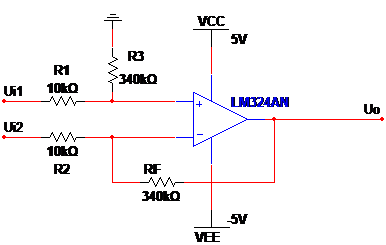电路的放大倍数A=R2/RF=33倍。正常情况下应保持R1=R2, R3=RF.
利用Multisim仿真差分放大电路如图所示，电压放大倍数约33倍，输入信号设定为峰峰值20mv，频率100hz的正弦信号，则预计输出信号为峰峰值660mv，频率100hz的正弦信号。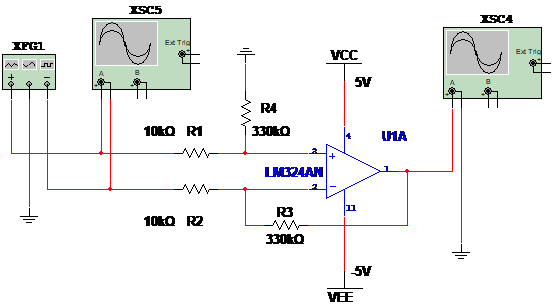差分放大仿真电路输入信号波形如图所示，峰峰值为20mv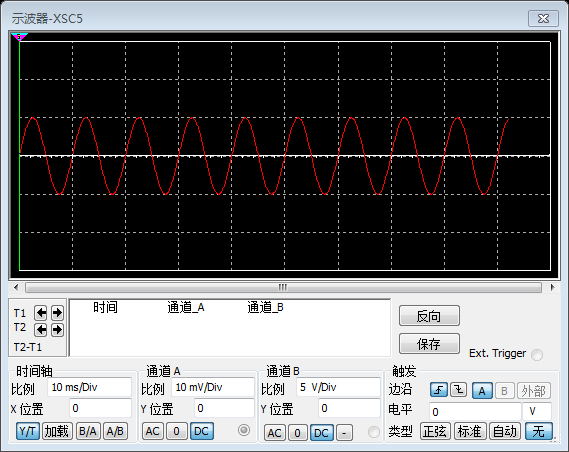差分放大仿真电路输出信号波形如图所示，峰峰值约为658.6mv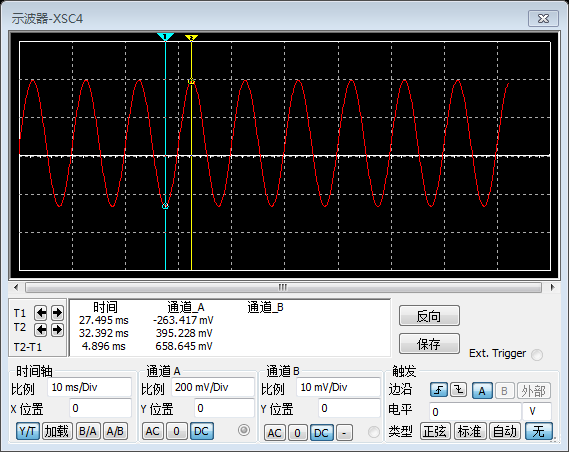2：带通滤波电路 带通滤波器（band-pass filter）是一个允许特定频段的波通过同时屏蔽其他频段的设备，在小信号处理电路中可以使我们所需求的那部分信号通过，从而更方便的处理有效信号。 例：设定带通滤波电路中心频率固定在1Hz。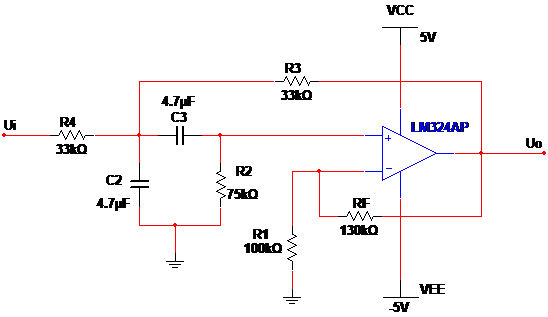电压放大倍数A=A0/3-A0=3.29倍，A0=1+RF/R1=2.3,中心频率为f0=1/2ΠRC=1.03HZ.为了防止自激震荡应使RF<2R1.
利用Multisim仿真带通滤波电路如图所示，通滤波仿真电路中心频率设为 f0=1HZ,电压放大倍数约3.3倍。输入信号设定为偏移量为1v，峰峰值500mv，频率1.2Hz的正弦信号并且伴随峰峰值不超过400mv的热噪声，经过带通滤波电路后预计输出信号为峰峰值1.65v，频率1.2Hz的正弦信号。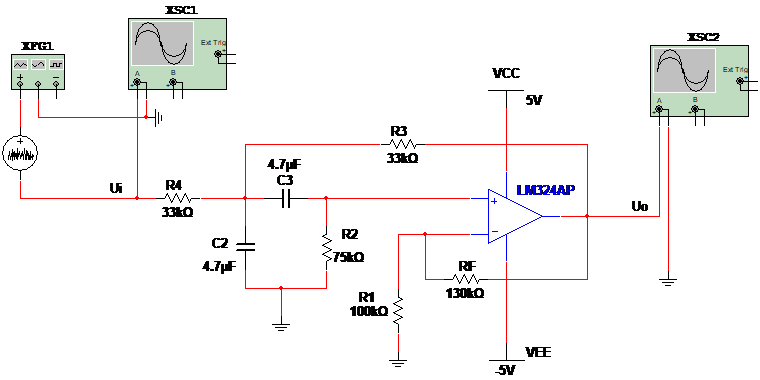带通滤波仿真电路输入信号波形如图所示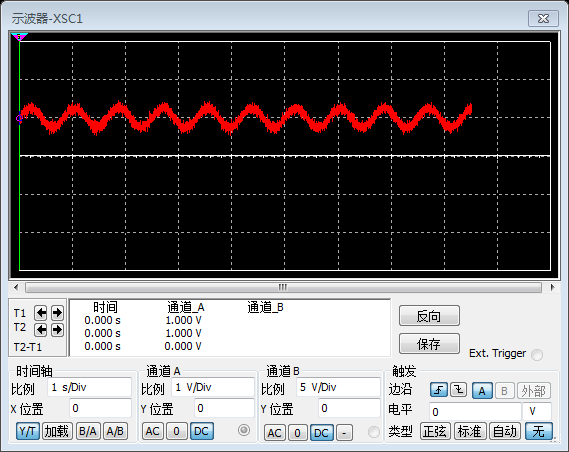带通滤波仿真电路输出信号波形如图所示 ，输出电压峰峰值约1.6v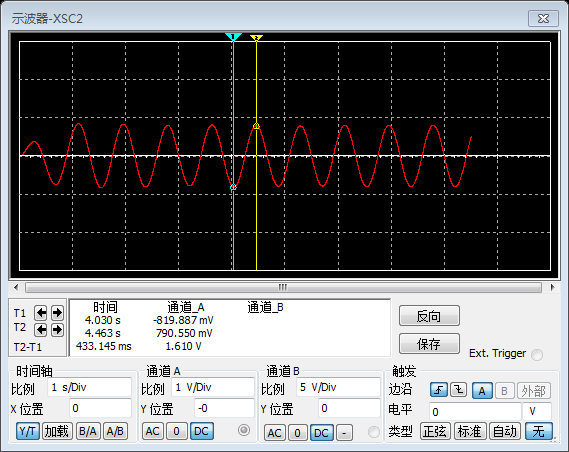3：加法电路 加法电路主要用来小波电压值。由于某些控制器不能采集负电压，因此需要将中心电压为0v的小信号与直流信号叠加，使输出电压均为正值，便于主控的采集。 例：设置最大采集电压为3.3v，因此设置信号调理电路输出的小波信号峰峰值最大不超过2v，中心电压为1.6v。如图所示，取R2=R3=R=200K,RF=10K,R1=10K,则R+=R/2=100K,U0=(1+RF/R1)((R+/R)1.6+R+/RUi)=1.6+Ui。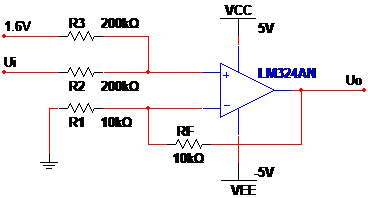利用Multisim仿真加法电路如图所示，一端输入信号Ui设定为峰峰值为2v，频率1.2Hz的正弦信号，另一端输入信号设定为1.6v的直流电压源，则预计输出信号为偏移量为1.6v，峰峰值2v的正弦信号。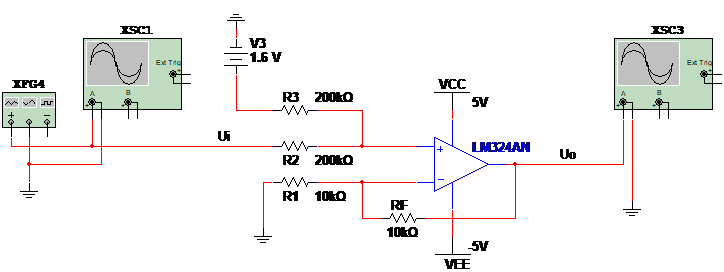加法仿真电路输入信号波形如图所示，峰峰值为2v的正弦信号加法仿真电路输出信号波形如图所示，输出电压为偏移量为1.6v，峰峰值2v的正弦信号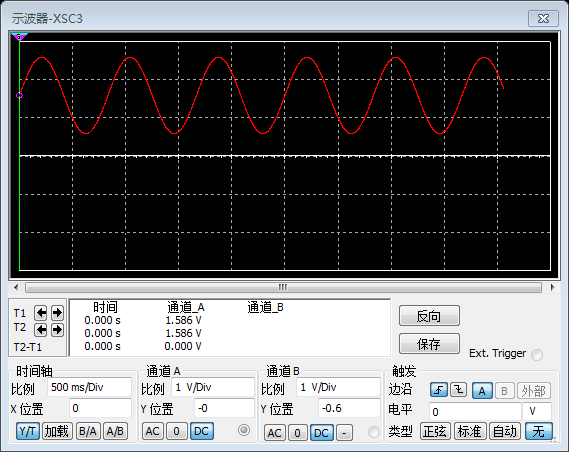4：电压跟随器
因为普通阻容滤波电路的输出阻抗比较高，与加法电路直接耦合，会使阻容滤波电路输出电压因为分压而发生衰减，无法实现预期功能，所以级联电压跟随器，达到阻抗匹配的目的 如图：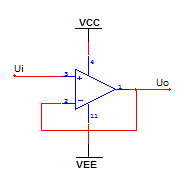注：本文中放大电路所用芯片均为LM324,M324系列器件带有真差动输入的四运算放大器，具有真正的差分输入。与单电源应用场合的标准运算放大器相比，它们有一些显著优点。该四放大器可以工作在低到3.0伏或者高到32伏的电源下，静态电流为MC1741的静态电流的五分之一。共模输入范围包括负电源，因而消除了在许多应用场合中采用外部偏置元件的必要性。 这几种电路使我们处理信号时时常能用到而且效果不错，在处理微弱信号上也有很强的能力，需要我们自己根据实际情况来搭配使用。还有许多经典电路在这就不一一写出了。 大家要是有好的方法，可以交流。
展开全文• 我总结了有以下几种利用matlab消除基线漂移的方法。 detrend函数 这是MATLAB内置系统函数，只能用于消除线性趋势（基线），不适用于消除非线性趋势，语法规则为： y = detrend(x) x：含有基线干扰的信号。 y：去除...
某些数字信号中会含有基线干扰信号（低频噪音），会对信号分析产生不利影响，如图1所示。需要通过预处理消除信号基线。图1 含有基线干扰的信号

我总结了有以下几种利用matlab消除基线漂移的方法。
detrend函数 这是MATLAB内置系统函数，只能用于消除线性趋势（基线），不适用于消除非线性趋势，语法规则为： y = detrend(x) x：含有基线干扰的信号。 y：去除基线干扰后的信号。 y = detrend(x,‘constant’) 减去信号的均值（修正零漂），等同于x-mean(x)。 y = detrend(x,‘linear’,bp) 分段消除时间序列中的线性趋势项,bp为分段点向量，分段点的值由其两端的线段共享。分段点的位置如下图所示。 信号处理中去除基线（baseline）干扰的几种方法图2 分段点位置

Examples1 输入： sig = [0 1 -2 1 0 1 -2 1 0]; % signal with no linear trend trend = [0 1 2 3 4 3 2 1 0]; % two-segment linear trend x = sig+trend; % signal with added trend y = detrend(x,‘linear’,5) % breakpoint at 5th element 输出： y = -0.0000 1.0000 -2.0000 1.0000 0.0000 1.0000 -2.0000 1.0000 -0.0000 Examples2 图3为两段含基线漂移的心电信号样本，第一段有向上的线性趋势，可直接去除： dt_ecgl = detrend(ecgl); 第二段有上下波动，接近分段线性，但断点不明显，不适用detrend函数去除。可以用下面描述的第2种方法：多项式拟合法去除。图3 去除基线前

多项式拟合 多项式拟合法是首先对含有线性趋势的信号进行多项式拟合，将基线拟合出来，然后把原始信号减去拟合信号，得到去除基线的信号。图4为用6阶多项式去除基线后的心电信号。 信号处理中去除基线（baseline）干扰的几种方法图4 去除基线后

示例程序（matlab）： opol = 6; [p,s,mu] = polyfit(t,ecgnl,opol); f_y = polyval(p,t,[],mu); dt_ecgnl = ecgnl - f_y;
BEADS算法 这是一个MATLAB第三方工具箱，全称：Baseline Estimation And Denoising w/ Sparsity。 点击这里可以查看详细介绍，作者原来是用于处理色谱信号，但是该算法也可用于处理其他含有基线干扰的信号。该算法的主要作用在于基线修正、降噪、仪器零漂修正功能。有一个使用的限制条件是被处理信号必须是大多数峰值是正的稀疏信号。算法效果见图5所示。图5 BEADS算法
  小波算法 通过小波变换的多尺度分解，在分解的低频系数中可观察到信号的基线趋势。再将原始信号减去基线即可。  经验模态分解（EMD） EMD方法认为任何信号都可以分解为若干个不同的本征模态函数，和一个残余量稳态量。其中各个本征模态函数反映了信号的局部特性,残余量反映了信号的趋势或均值。EMD法采用“筛”选的方法从原始信号中将残余量分离出来。
reference:  http://cn.mathworks.com/matlabcentral/fileexchange/49974-beads–baseline-estimation-and-denoising-w–sparsity–chromatogram-signals-?s_tid=AO_FX_info
展开全文matlab
• 通过研究3AM信号数字化解调的算法，给出相应的解调原理、公式推导以及系统模块；采用Matlab对一段信号采用3种方法分别进行仿真解调，并对结果进行比较。在原理分析与仿真结果的基础上，通过比较获得3种方法各自的...
• matlab 信号处理中去除基线（baseline）干扰的几种方法 http://blog.sina.com.cn/s/blog_619c66670102wm2u.html
matlab 信号处理中去除基线（baseline）干扰的几种方法 http://blog.sina.com.cn/s/blog_619c66670102wm2u.html
展开全文• 综述了国内外在涡街流量计研究中的信号处理方法。...涡街流量计信号处理几种有代表性的方法。 在此基础上还介绍了作者自行研制的以数字信号处理器为处理核心的涡街流量计信 号处理系统的软、硬件框图。
• 几种常用信号平滑去噪的方法（附Matlab代码）1 滑动平均法1.0 移动平均法的方法原理1.1 matlab内自带函数实现移动平均法1.2 利用卷积函数conv()实现移动平均法1.3 利用filter滤波函数实现移动平均法1.4 移动平均的...


几种常用信号平滑去噪的方法（附Matlab代码）
1 滑动平均法1.0 移动平均法的方法原理1.1 matlab内自带函数实现移动平均法1.2 利用卷积函数conv()实现移动平均法1.3 利用filter滤波函数实现移动平均法1.4 移动平均的幅频响应1.5 时域和频域的转换关系
2 Savitzky-Golay法2.1 Savitzky-Golay法的方法原理2.2 Savitzky-Golay法的matlab实现2.3 Savitzky-Golay法的幅频响应
4 其它一些FIR滤波器实现光滑去噪4.1 FIR和IIR的区别4.2 利用Matlab构建FIR滤波器
5 IIR滤波器实现光滑去噪6 小波去噪方法

2020年8月更新：增加一个时域和频域的转换关系图，增加相应小节1.5 2021年8月更新：增加第6章小波去噪的内容
信号在实际测量中，难免会混入各种噪声。通常我们希望去除高频的随机噪声，或者是偏离正常测量太大的离群误差，以获得低频的测量数据。下面介绍几种常用的信号平滑去噪的方法。
还是惯例声明：本人没有相关的工程应用经验，只是纯粹对相关算法感兴趣才写此博客。所以如果有错误，欢迎在评论区指正，不胜感激。本文主要关注于算法的实现，对于实际应用等问题本人没有任何经验，所以也不再涉及。
1 滑动平均法
本章参考目录 1《数字信号处理》-胡广书 2《Digital Signal Processing - A Practical Guide For Engineers and Scientists》 - Steven W.Smith
1.0 移动平均法的方法原理
作为开篇第一个方法，会夹带一些数字信号处理的基本方法，可能会导致篇幅比较啰嗦，之后几章我会尽量挑重点的讲解。
滑动平均法（moving average）也叫做移动平均法、平均法、移动平均值滤波法等等，是一种时间域思想上的信号光滑方法。算法思路为，将该点附近的采样点做算数平均，作为这个点光滑后的值。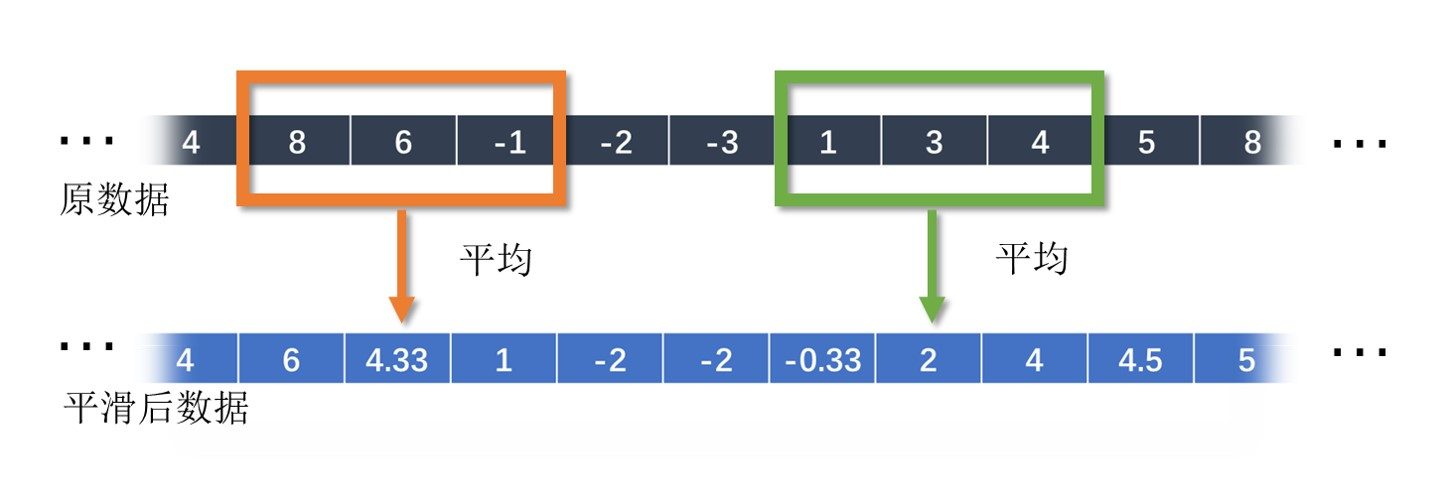一般窗口为对称窗口，防止出现相位偏差。窗口一般为奇数。 以3点平均（窗口长度为3）公式为例，原数据为x，平滑后的数据为y：

y

(

n

)

=

1

/

3

∗

(

x

(

n

−

1

)

+

x

(

n

)

+

x

(

n

+

1

)

)

y(n)=1/3*( x(n-1)+x(n)+x(n+1) )

对y(n)和y(n+1)相减，可以得到另一种计算形式：

y

(

n

+

1

)

=

y

(

n

)

−

1

3

x

(

n

−

1

)

+

1

3

x

(

n

+

2

)

y(n+1)=y(n)-\frac{1}{3}x(n-1)+\frac{1}{3}x(n+2)

当然这两者都是等价的。
1.1 matlab内自带函数实现移动平均法
matlab有两个函数实现滑动平均法，一个是smoothdata()函数，一个是movmean()函数。 以窗口长度为5为例，smoothdata()函数调用方法为：
y = smoothdata( x , 'movmean' , 5 );

但是这个smoothdata函数实际上是调用了movmean()函数。所以如果直接使用的话，直接用movmean()会更快。 movmean()函数的调用方法为：
y = movmean( x , 5 );

下面以一个加噪声的正弦信号为例：
%移动平均滤波
clear
clc
close all

N_window = 5;%窗口长度(最好为奇数）
t = 0:0.1:10;
A = cos(2*pi*0.5*t)+0.3*rand(size(t));
B1 = movmean(A,N_window);
figure(1)
plot(t,A,t,B1)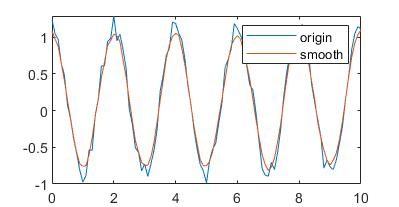1.2 利用卷积函数conv()实现移动平均法
根据之前的公式，我们可以看到

y

(

n

)

=

1

/

3

∗

(

x

(

n

−

1

)

+

x

(

n

)

+

x

(

n

+

1

)

)

y(n)=1/3*( x(n-1)+x(n)+x(n+1) )

就相当于一个对x和向量[1/3 1/3 1/3]做卷积。 可以验证：
N_window = 5;%窗口长度(最好为奇数）
t = 0:0.25:10;%时间
A = cos(2*pi*0.5*t)+0.3*rand(size(t));
B1 = movmean(A,N_window);

F_average = 1/N_window*ones(1,N_window);%构建卷积核
B2 = conv(A,F_average,'same');%利用卷积的方法计算
figure(2)
plot(t,B1,t,B2)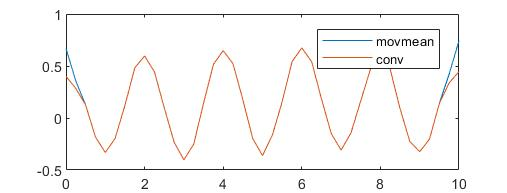中间部分两者完全一致，但是两端有所差别。主要是因为，movmean()函数在处理边缘时，采用减小窗口的方式，而conv()相当于在两端补零。所以如何处理边缘也是值得注意的。
1.3 利用filter滤波函数实现移动平均法
首先介绍一下Z变换。以向前的滑动平均为例（这里中间值不是n而是n+1，所以相位会移动）。

y

(

n

)

=

1

/

3

∗

(

x

(

n

)

+

x

(

n

+

1

)

+

x

(

n

+

2

)

)

y(n)=1/3*( x(n)+x(n+1)+x(n+2) )

它的Z变换可以简单的理解为，把x(n+k)替换为z^(-k)，即

H

(

z

)

=

1

3

(

z

0

+

z

−

1

+

z

−

2

)

H(z)=\frac{1}{3}(z^{0}+z^{-1}+z^{-2})

因此对于filter滤波函数，输入的格式为：
y = filter(b,a,x)

其中b和a的定义为：

H

(

z

)

=

b

1

+

b

2

⋅

z

−

1

+

b

3

⋅

z

−

2

+

⋯

+

b

n

⋅

z

−

n

+

1

1

+

a

2

⋅

z

−

1

+

a

3

⋅

z

−

2

+

⋯

+

a

m

⋅

z

−

m

+

1

H(z)=\frac{b_1+b_2\cdot z^{-1}+b_3\cdot z^{-2}+\cdots +b_{n}\cdot z^{-n+1}}{1+a_2\cdot z^{-1}+a_3\cdot z^{-2}+\cdots +a_{m}\cdot z^{-m+1}}

其中a1必须为1。所以对应的移动平均滤波可以表示为：
y = filter( [1/5,1/5,1/5,1/5,1/5] ,  , x );

它和下面代码的是等价的（在边缘上的处理方式有所不同）
y = movmean( x , [4,0] );

代表有偏移的滑动平均滤波，4是向后4个点的意思，0是向前0个点的意思。
因为 filter滤波器使用有偏移的向后滤波。滤波后，相位会发生改变。所以通常采用零相位滤波器进行滤波，matlab内的函数为filtfilt()。原理从函数名字上就可以体现出来，就是先正常滤波，之后再将信号从后向前再次滤波。正滤一遍反滤一遍，使得相位偏移等于0。这个之后再IIR滤波器会讲一下。
1.4 移动平均的幅频响应
幅频响应可以通过之前1.3得到的H(z)函数来得到，在单位圆上采样，也就是把z替换为e^iw。 以中心窗口为例，

y

(

n

)

=

1

3

(

x

(

n

−

1

)

+

x

(

n

)

+

x

(

n

+

1

)

)

y(n)=\frac{1}{3} ( x(n-1)+x(n)+x(n+1) )

H

(

i

w

)

=

1

3

(

exp

⁡

(

i

w

)

1

+

exp

⁡

(

i

w

)

0

+

exp

⁡

(

i

w

)

−

1

)

H(iw)=\frac{1}{3}(\exp(iw)^{1}+\exp(iw)^{0}+\exp(iw)^{-1})

H(iw)的绝对值就是该滤波方法的幅频响应。以3点滤波为例，matlab代码为：
%计算频率响应
N_window = 3;
w = linspace(0,pi,400);
H_iw = zeros(N_window,length(w));
n=0;
for k = -(N_window-1)/2:1:(N_window-1)/2
n = n+1;
H_iw(n,:) =1/3* exp(w.*1i).^(-k);%公式(e^iw)^(-k)
end
H_iw_Sum = abs(sum(H_iw,1));%相加

figure()
plot(w/2/pi,H_iw_Sum)

由于H变换在单位圆上的特性相当于傅里叶变换，所以上面代码等效于下面计算方法：
%计算频率响应
N_window = 3;
Y=zeros(400,1);
Y(1:N_window) = 1/3;%设置滤波器
Y_F = fft(Y);
figure()
plot(linspace(0,0.5,200),abs(Y_F(1:200)));

matlab也有自带的函数来看频率特性，freqz()，推荐使用这种。
其中，归一化频率等于信号频率除以采样频率f/Fs，采样频率等于时间采样间隔的倒数1/dt。对比不同窗口长度的幅频响应，可以看到： 1平均所采用的点数越多，高频信号的滤波效果越好。 2 3点平均对于1/3频率的信号滤波效果最好，5点平均对1/5和2/5频率的信号滤波效果最好。所以根据这个特性，一方面我们要好好利用，一方面也要避免其影响。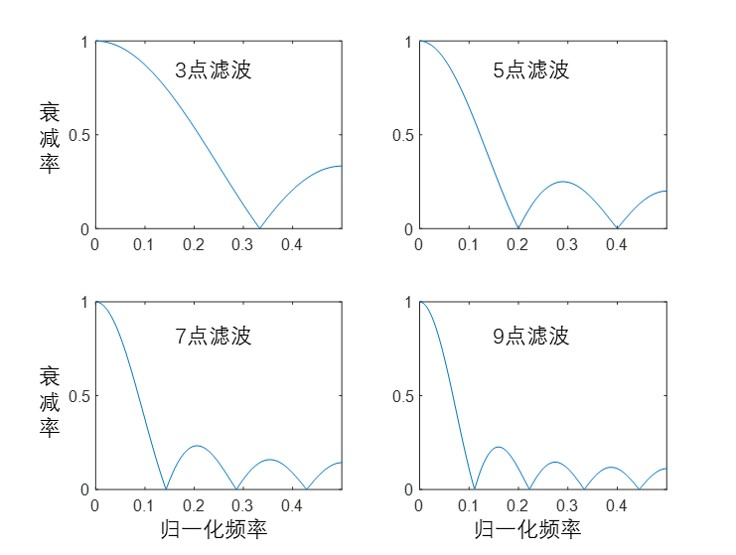举个应用的例子，比如你的采样频率为10hz，采样3点滑动平均滤波，但是你的信号在3.3hz左右，你就会发现你的信号被过滤掉了，只留下了噪声。
反之，以美国近期的疫情为例，疫情的采样频率为1天一采样，而且显示出很强的7日一周期的特性（病毒也要过周末）。所以，为了消除这个归一化频率为1/7的噪声影响，采样7点的滑动平均滤波。可以看到所有以7天为一变化的信号分量全部被消除掉了。 （下面这个图经常被引用，但是很少有人思考为什么用7天平均方法来平滑数据。）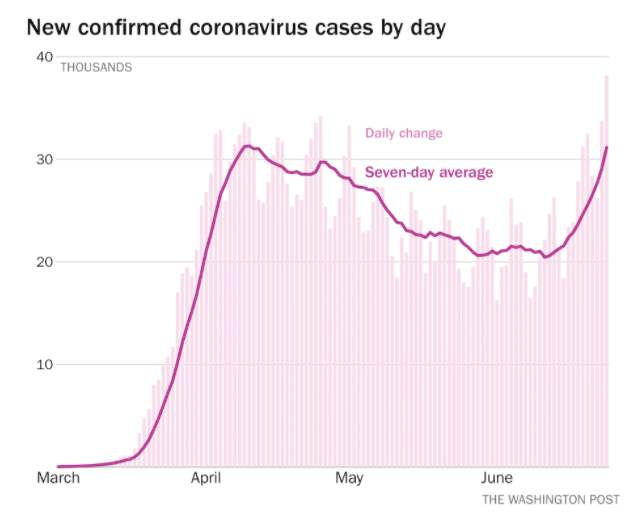回到原本的幅频特性问题上。当点数较少的时候，比如3个点，高频滤波效果并不是很好。所以当取的点数比较少的时候，需要平滑完一遍之后再平滑一遍，直到满意为止。这个原理也可以通过幅频特性来解释，多次平滑相当于乘了多个H(z)。对于平滑2次，它的图像也就是abs(sum(H_iw,1).*sum(H_iw,1))；对于平滑4次，它的图像相当于乘以四个sum(H_iw,1)。（注：因为时域上的卷积等于频域上的乘积，多次卷积对应着多次乘积。）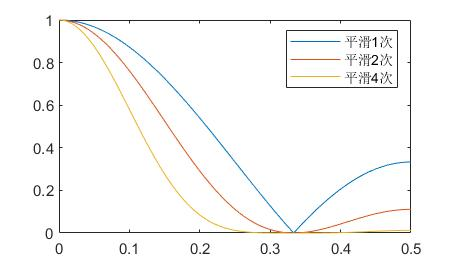可以看到，多次平滑之后，高频的衰减非常明显。这也就是说，即使只有3个点平均，多次平滑之后也可以等效为一个较好的低通滤波器。
所以总结一下，移动平均滤波拥有保低频滤高频的特点，而且对于特点频率的滤波具有良好的效果。但是缺点是所有频率分量的信号都会有不同程度衰减。
1.5 时域和频域的转换关系
额外补充一部分小内容，可能前面有些概念加入的太突然。很多人可能觉得之前时域上的平均法非常好理解，为什么突然加入幅频特性图，又是Z变换又是fft的。
其实时域上的滤波和频域上的滤波是可以互相转换，且一一对应的。也就是时域上的卷积等于频域上的乘积。 下图为3点移动平均滤波法，时域和频域的转换关系：同样的滤波操作，可以用时域公式：

y

(

n

)

=

1

3

(

x

(

n

−

1

)

+

x

(

n

)

+

x

(

n

+

1

)

)

y(n)=\frac{1}{3} ( x(n-1)+x(n)+x(n+1) )

，进行描述。也可以用频域上，滤波器的幅频特性进行描述。
虽然前面的 movmean()或者conv()等函数都是用时域实现的信号滤波，但是同样也可以完全在频域上实现。采用ifft(fft(x).*fft(F))实现的滤波效果，和完全时域上的滤波效果是等价的。
下面是展示了窗口长度为3的平滑滤波，从时域上和频域上对信号进行滤波的对比：
%实验，检验频域和时域的一致性
%以3点滤波为例
clear
clc
close all

N_window = 3;%窗口长度(最好为奇数）
t = 0:0.1:10;
A = cos(2*pi*0.3*t)+0.1*cos(2*pi*5*t)+0.2*randn(size(t));

F_average = 1/N_window*ones(1,N_window);%创建滤波器
B2 = conv(A,F_average,'same');%利用卷积的方法计算

figure(1)
plot(t,A,'k','linewidth',0.8)

%计算原信号的fft
A_fft=fft(A);
%构建频域上的滤波器
F_average2=zeros(size(t));%长度与x相同，为了后面.*运算
F_average2(1:(N_window-1)/2+1) = 1/N_window;
F_average2(end-(N_window-1)/2+1:end) = 1/N_window;%前后设置对称，使得相位不变
F_Fft = fft(F_average2);

figure(2)
subplot(1,2,1)
plot(linspace(0,1,length(t)),abs(A_fft),'linewidth',1);
subplot(1,2,2)
plot(linspace(0,1,length(t)),abs(F_Fft),'linewidth',1);

%进行反逆变换
B3=ifft(A_fft.*F_Fft);

figure()
plot( t,B2,t,B3 )%对比时域操作和频域操作的差异

这也意味着你也可以在频域上操作，实现想要的滤波。比如想要低频通过高频衰减，就把fft后的信号，高频部分强行等于0即可。比如想要消除某个频率的信号（陷波），就令fft后那个信号的频率等于0即可。同理，想要把振幅衰减1/2，就在对应频域上乘以0.5。
2 Savitzky-Golay法
本章参考目录： 1《数字信号处理》-胡广书 2 Savitzky-Golay平滑滤波器的最小二乘拟合原理综述 https://wenku.baidu.com/view/b63017eed0d233d4b04e690e.html?fr=search
2.1 Savitzky-Golay法的方法原理
Savitzky-Golay法，又叫做平滑滤波器，最著名的就是5点3次滤波器。这是一种基于时间域上的多项式拟合，来消除噪声的方法。
以简单的5点2次构造方法为例，介绍一下基本的求解系数的方法： 首先选取5个点x[-2],x[-1],x,x,x，根据这5个点，构造一条2次抛物线：

f

(

i

)

=

a

0

+

a

1

⋅

i

+

a

2

⋅

i

2

f(i)=a_{0}+a_{1} \cdot i+a_{2} \cdot i^2

这里i=-2,-1,0,1,2。因此，我们需要寻找最优的a0,a1,a2，使得最小二乘拟合最小。最小二乘拟合的函数为：

E

=

∑

(

f

(

i

)

−

x

(

−

2

)

)

2

=

(

f

(

−

2

)

−

x

(

−

2

)

)

2

+

(

f

(

−

1

)

−

x

(

−

1

)

)

2

+

+

(

f

(

0

)

−

x

(

0

)

)

2

+

(

f

(

1

)

−

x

(

1

)

)

2

+

(

f

(

2

)

−

x

(

2

)

)

2

E=\sum{(f(i)-x(-2))^2}\\ =(f(-2)-x(-2))^2+(f(-1)-x(-1))^2+\\ +(f(0)-x(0))^2+(f(1)-x(1))^2+(f(2)-x(2))^2

之后希望最小二乘E最小，我们使得其导数等于0，也就是

∂

E

∂

a

p

=

0

\frac{\partial E}{\partial a_p}=0

上面式子中，a0、a1和a2三个偏导数，可以得到3个方程。联立，即可求得a0、a1和a2。 对于无相位差的滤波，我们希望窗口是对称的。也就是说，我们希望用5个点，去估计f的值。因此我们需要的只有a0，因为：

f

(

0

)

=

a

0

+

a

1

⋅

0

+

a

2

⋅

0

=

a

0

f(0)=a_{0}+a_{1} \cdot 0+a_{2} \cdot 0=a_{0}

这里借助Mathematica的符号运算进行编程求解，程序如下：
Clear["Global*"]
M = 5;(*M个点*)
P = 2;(*P次*)

Mk = (M - 1)/2;
f[i_] = Sum[a[k]*i^k, {k, 0, P}]
En = Sum[(f[k] - x[k])^2, {k, -Mk, Mk}];
DEn = D[En, {Array[a, P + 1, {0, P}]}];
s = Solve[DEn == 0, Array[a, P + 1, {0, P}]];
s[][]

可以得到结果：
a -> 1/35 (-3 x[-2] + 12 x[-1] + 17 x + 12 x - 3 x)

对于经典的5点3次平滑结果，只需要把上面代码P改为3即可，恰好结果相同：
a -> 1/35 (-3 x[-2] + 12 x[-1] + 17 x + 12 x - 3 x)

Savitzky-Golay法可以看做是移动平均法的推广。因为当次数P等于1时，Savitzky-Golay法退化为求解平均值的形态。当然，它也可以视为一种加权平均。其它加权平均比如高斯加权等等，可以在smoothdata函数里的帮助文档看到，就不细说了。
除此之外，也可以直接用matlab中的sgolay()函数，直接得到系数，还是以5点3次为例：
M=5;%5点
P=3;%3次
b = sgolay( P , M );
a0 = b((M+1)/2,:)

2.2 Savitzky-Golay法的matlab实现
matlab中实现方法和第一章中滑动滤波的方法相似。 利用matlab自带的smoothdata(A,‘sgolay’)函数就可以实现Savitzky-Golay法滤波。但是，该函数只支持N点2次的滤波（2.1阶证明了5点2次和5点3次的a0滤波系数相同，但是其他点数则不相同，这一点一定注意）。
如果想得到不同点数不同次数的公式，参考2.1中的sgolay()函数，可以得到不同的系数。下面代码演示了一个利用matlab自带的5点2阶插值和自己编程实现的5点3次插值，其中自己编程的部分采用了1.2节部分的卷积运算，和2.1节介绍的matlab自带函数sgolay()。
%sgolay滤波
clear
clc
close all

N_window = 5;%窗口长度(最好为奇数）

t = 0:0.1:10;
A = cos(2*pi*0.5*t)+0.4*rand(size(t));

%matlab自带的5点2次插值
B1 = smoothdata(A,'sgolay' ,N_window);
figure(1)
plot(t,A,t,B1)

%5点3次插值
B2 = smooth_SG_hyh(A,5,3,1);
figure(2)
plot(t,B1,t,B2)

function y_new = smooth_SG_hyh(y,M,P,n)
%M点P次插值
%M为窗口长度
%P为拟合阶数
%n为光滑n次

m = length(y);
N_window = M;%窗口长度
b = sgolay( P , N_window );
F_SG = b((N_window+1)/2,:);%5点3次插值
y_new = y;
for k=1:n
y_new2 = wextend(1,'sym',y_new,(N_window-1)/2);%镜像延拓
y_new2 = conv(y_new2,F_SG,'same');%利用卷积的方法计算
y_new = wkeep1(y_new2,m);%中间截断
end

end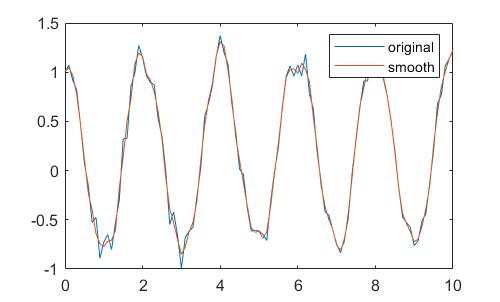2.3 Savitzky-Golay法的幅频响应
利用之前1.4的内容，可以求出Savitzky-Golay法的幅频响应。
%频率响应对比
[w,A_W_5_3] = A_W_SG_hyh(5,3);
[w,A_W_5_1] = A_W_SG_hyh(5,1);
figure()
plot(w,A_W_5_1,w,A_W_5_3)

%频率响应对比
[w,A_W_7_3] = A_W_SG_hyh(7,3);
[w,A_W_7_1] = A_W_SG_hyh(7,1);
figure()
plot(w,A_W_7_1,w,A_W_7_3)
ylim([0,1])

function [w_2p,H_iw_Sum] = A_W_SG_hyh(M,P)
N_window=M;
b = sgolay( P , N_window );
F_SG = b((N_window+1)/2,:);%5点3次插值

w = linspace(0,pi,400);
H_iw = zeros(N_window,length(w));
n=0;
for k = -(N_window-1)/2:1:(N_window-1)/2
n = n+1;
H_iw(n,:) =F_SG(n)* exp(w.*1i).^(-k);%公式(e^iw)^(-k)
end
H_iw_Sum = abs(sum(H_iw,1));%相加
w_2p = w/2/pi;
end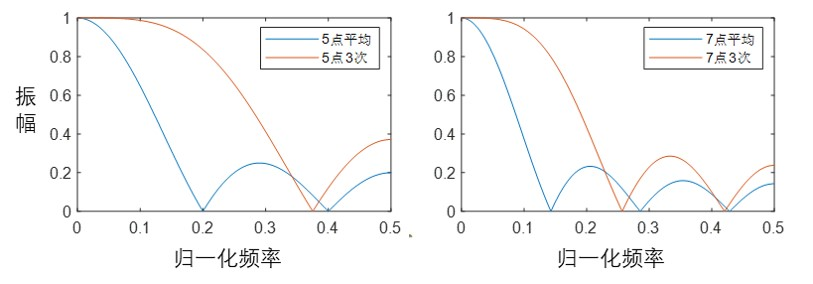可以看到，当拟合的次数增加，该方法对低频的滤波效果变差。所以和平均法相比，5点3次方法的频率特性与3点移动平均比较相似。因此，5点3次方法适用于噪声频率比较高，信号频率比较低（说的都是归一化频率，也就是和采样频率之比）的情况。相比较相同频率效果的平均法，Savitzky-Golay法用了更多的点，在时域上保留了更好信息。
3 处理离群值（粗大误差）的方法
离群值是指在测量值中，出现了一些反常的值，这个反常值与附近的其它正常值差别非常大。
常用的方法有利用

3

σ

3 \sigma

本章参考目录： 1 离群值处理方法 https://blog.csdn.net/zjz199303/article/details/79135530 2 常用去除离群值的算法 https://blog.csdn.net/dulingwen/article/details/97006884
3.1 中位值法
中位值法，也叫移动中位数法、中值滤波法等。其思想是将窗口内的中位数作为输出结果，如下图所示：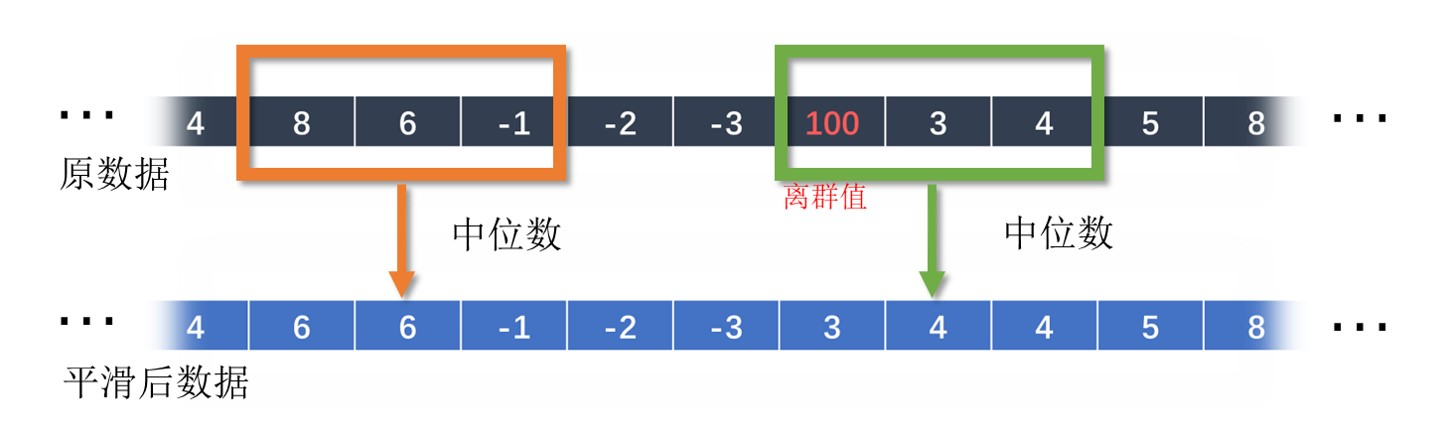优点是，在数据采样点密集，且比较平滑的情况下，中位数法可以很好地剔除离群值。缺点是不适用于噪声较大的情况。而且平滑之后，数据光滑度不足。经过中位值法处理之后，极值点会丢失。
matlab中自带的movmedian()函数可以实现中位值法滤波。
clear
clc
close all

%离群值的删除
t = 0:0.05:10;
A = sin(t);
Ri = randi(length(t),10,1);
A(Ri) = A(Ri)*2;

%移动中位数，窗口设置为7
B1 = movmedian(A,7);
figure(1)
plot(t,A,t,B1)

下图可以看到中位值法对于离群值处理的结果。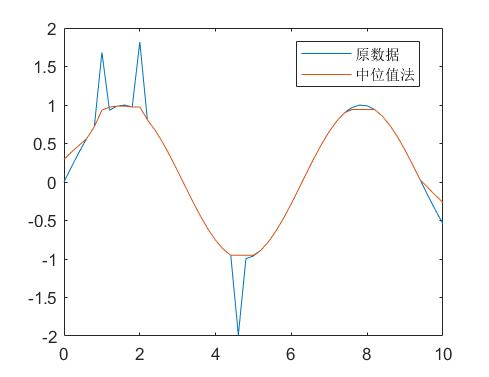虽然中值方法能够将所有离群值筛除，但是在8s处的波峰位置，极值点被不属于中位数，所以被消除了。在2s处，由于噪声比较复杂，所以出现了信号不光滑的现象（这里如果加上高频噪声，不光滑现象会更明显）。
标准差法又叫做

3

σ

3 \sigma

法。目的是规定一个数据波动阈值，当数据超过这个阈值的时候，便认为该数据离群。这个方法阈值的选取方法，采用窗口数据的3倍标准差。
clear
clc
close all

%离群值的删除
t = 0:0.06:10;
A = sin(t)+0.1*rand(size(t));

Ri = randi(length(t),4,1);
A2 = A;
A2(Ri) = A(Ri)*3;

figure(3)
B2 = filloutliers(A2,'linear','movmean',11);%标准差法

subplot(3,1,1)
plot(t,A2)
YL = ylim;
subplot(3,1,2)
plot(t,B2)
ylim(YL)
subplot(3,1,3)
plot(t,B3)
ylim(YL)

下图可以看到，原本4个离群值，标准差法只找到了1个，但是MAD方法能够做到全部找到，说明MAD方法比标准差法的效果更好，一般更推荐用MAD方法。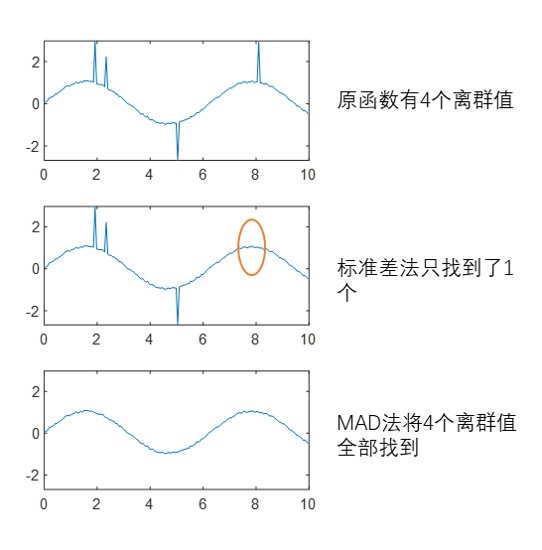3.3 Matlab中其它离群值消除方法
matlab自带的函数smoothdata还有两种方法，可以兼顾去噪和去除离群噪声，一个是’rlowess’ 方法，一个是’rloess’ 方法。下面以’rloess’ 方法为例：
t = 0:0.06:10;
A = sin(t)+0.2*rand(size(t));

Ri = randi(length(t),4,1);
A2=A;
A2(Ri) = A(Ri)*3;
figure(4)
B4 = smoothdata(A2,'rloess',8) ;
plot(t,A2,t,B4)
legend('原函数','光滑')

下图可以看到，数据在光滑的同时，顺便也把离群值去掉了。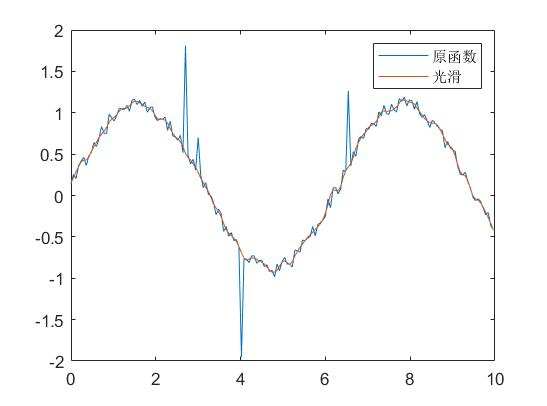4 其它一些FIR滤波器实现光滑去噪
本章参考： Matlab数字滤波入门 https://zhuanlan.zhihu.com/p/65483011
4.1 FIR和IIR的区别
FIR滤波器也叫做 有限冲击响应滤波器。和它相对的是IIR 无限冲击响应滤波器。在matlab里，有一张图可以比较直观。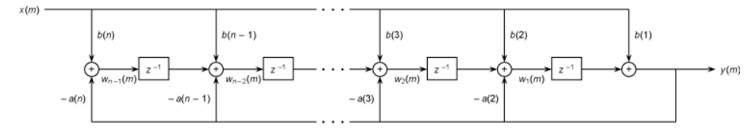对于FIR滤波器，用到的数据只有输入项b，输出项为1。但是对于IIR滤波器，不仅有输入项b（分子），还有输出项a（分母）。
对于FIR滤波器，由于只有分子b，所以可以用卷积conv()函数去进行滤波。但是对于IIR，就不能用卷积函数去计算。一般认为，IIR函数在相同阶数下，滤波效果要比FIR函数要好，但是有可能出现发散问题。
比如前面的移动平均滤波中的1.3章所介绍的，b=[1/3,1/3,1/3]，a=1。就属于一个典型的FIR滤波器。其中，conv(x,b)卷积方法相当于无相位偏移的中心滤波，

y

(

n

)

=

1

3

(

x

(

n

−

1

)

+

x

(

n

)

+

x

(

n

+

1

)

)

y(n)=\frac{1}{3} ( x(n-1)+x(n)+x(n+1) )

而filter(b,a,x)函数相当于有相位偏移的向后滤波

y

(

n

)

=

1

3

(

x

(

n

)

+

x

(

n

+

1

)

+

x

(

n

+

2

)

)

y(n)=\frac{1}{3} ( x(n)+x(n+1)+x(n+2) )

；
事实上，通过下面的等式，我们还可以构建一个等价的IIR滤波器：

H

(

z

)

=

1

3

(

z

0

+

z

−

1

+

z

−

2

)

=

1

3

1

−

z

−

3

1

−

z

−

1

H(z)=\frac{1}{3}(z^{0}+z^{-1}+z^{-2})=\frac{1}{3}\frac{1-z^{-3}}{1-z^{-1}}

此时b=[1,0,0,0,-1]，a=[1,-1]。
Fs=20;
t = 0:1/Fs:10;
A = cos(2*pi*0.3*t)+1*sin(2*pi*1.2*t)+0.8*rand(size(t));

b=[1/3,1/3,1/3];a=1;
B1 = filter(b,a,A);

b=1/3*[1,0,0,0,-1];a=[1,-1];
B2 = filter(b,a,A);

plot(t,B1,t,B2)

对比这两个滤波器，可以看到滤波效果是基本等价的。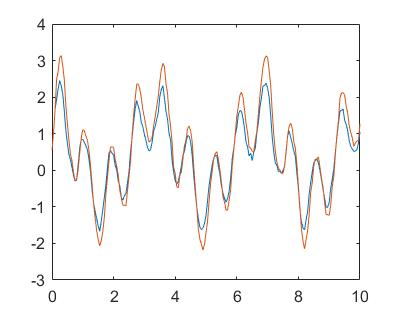4.2 利用Matlab构建FIR滤波器
回到FIR滤波器上，之前提到的时域角度提出的均值滤波器和Savitzky-Golay滤波器，都属于FIR滤波器。他们在频域上的表现并不能随意的控制。
如果我们希望构建一个滤波器，让它在频域上满足自己的要求，则可以用fir1()函数去构建。我这里只演示一个简单地，更专业的就不在这里献丑了。
比如，我的采样频率为10hz，我想提取的信号在1hz附近，而噪声则大于等于2hz。因此，需要构建一个低频通过，高频减弱的滤波器，使得在1.5hz以下的信号能够保留，1.5hz以上的信号删除。
于是用下面代码去构建，滤波器长度为31，保留0-0.15之内的信号。（注1：matlab的归一化信号不是0-0.5，而是0-1，所以对应的0.15需要乘以2倍，变成0.3）（注2：最小频率不能是0，必须大于0，所以用0.001代替）（注3：滤波器长度为31是为了演示，实际上不需要这么大的长度，滤波器长度大了的话计算量也会增大）
b = fir1(31,[0.001,0.3]);

构建的滤波器形状和频率特性为：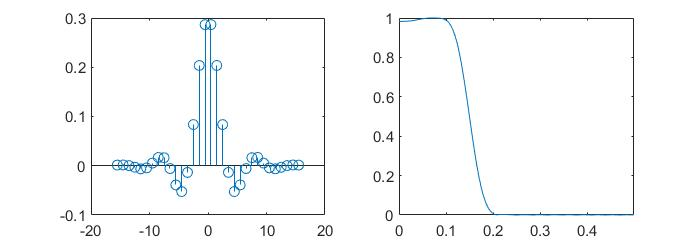滤波器形状很像sin(x)/x函数曲线。因为如果频率特性为理想的阶梯形状，则滤波器时域形态从数学上求解就是sin(x)/x函数，也被简写为sinc(x)函数。这种滤波器叫做sinc滤波器，由于其频域像一个矩形，也叫作矩形滤波器。特点就是低频完全通过，高频全部衰减为0，是一种理想的滤波器。
频率特性满足预期的设计。滤波效果如下：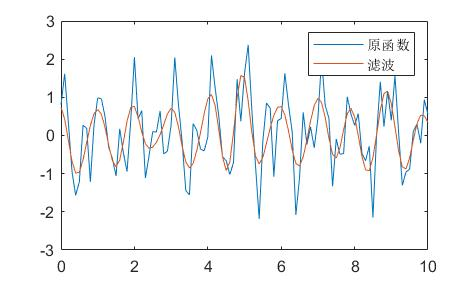附上上面的matlab代码
clear
clc
close all

Fs=10;
t = 0:1/Fs:10;
A = cos(2*pi*1*t)+0.8*sin(2*pi*2*t+0.5*randn(size(t)))+0.5*randn(size(t));

b = fir1(31,[0.001,0.3]);

figure()
subplot(1,2,1)
N=length(b);
stem(-(N-1)/2:1:(N-1)/2,b)%绘制滤波器形状
subplot(1,2,2)
[h,w]=freqz(b,1,512);%输出频率特性
plot(w/2/pi,abs(h))
xlim([0,0.5]);ylim([0,1])

B2 = conv(A,b,'same');%利用卷积的方法计算
%因为FIR滤波可以利用卷积方法代替，这两个等效的

figure()
plot(t,A,t,B2)
legend('原函数','滤波')

5 IIR滤波器实现光滑去噪
比较著名有巴特沃斯滤波器butter()和切比雪夫滤波器cheby1()。这里感觉还有地方没看懂，就不详细写了。matlab里可以通过designfilt()来自定义的构建滤波器。
以butter滤波器为例，采用零相位滤波，和第4.2节一样，希望保留0-0.15之内的信号。那么构建滤波器格式为：
[b,a] = butter(6,0.15*2,'low' );

b为分子，a为分母。6为6阶，代表滤波器的长度为6+1个点。'low’是低通滤波器的意思，0.15*2代表保留0-0.15之内的信号。得到的滤波器幅频响应曲线如下，可以看到能够满足要求。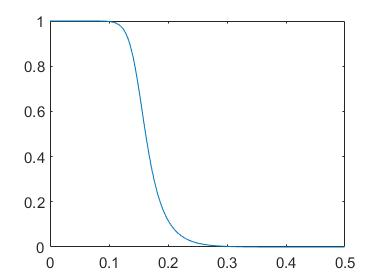利用butter滤波器进行光滑去噪的matlab代码如下：
%butter滤波器
clear
clc
close all

Fs = 10;
t = 0:1/Fs:10;
A = cos(2*pi*1*t)+0.8*sin(2*pi*2*t+0.5*randn(size(t)))+0.5*randn(size(t));
[b,a] = butter(6,0.15*2,'low' );
B = filtfilt(b,a,A);

figure()
[h,w]=freqz(b,a,512);
plot(w/2/pi,abs(h))
xlim([0,0.5]);ylim([0,1])

figure()
plot(t,A,t,B)
legend('原函数','滤波')

平滑后的曲线效果如下图。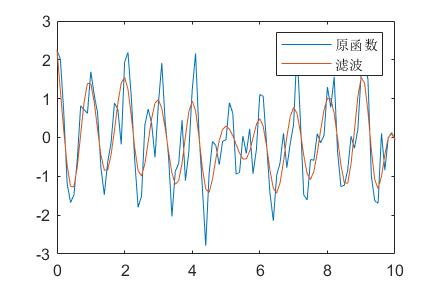6 小波去噪方法
小波去噪最近刚刚接触，这里也更新补充一下。后续具体原理和算法，我尽快补充上（又挖坑）。和传统去噪相比，其优势在于可以更好的提取出信号的主要部分，受噪声影响较小。
比如下面这个图，传统的移动平均去噪（也包括前面介绍的各种滤波器），由于在频域上相当于全局频域上的滤波，所以会出现：高频信号和噪声一块被滤掉了，中间频域去噪效果比较好，低频受噪声影响严重滤波不足 的现象。这就是全局滤波的缺点，按下葫芦浮起瓢，不能做到高频低频同时兼顾。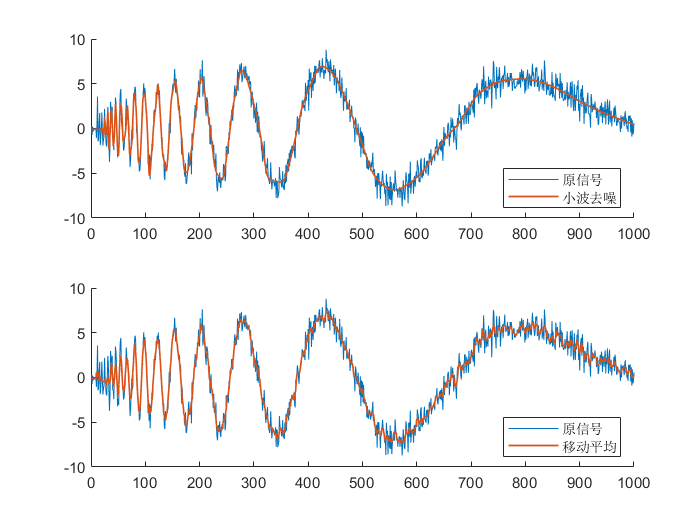相比较而言，小波去噪效果就好很多，不仅高频处保留了更多的高频信号信息，低频处的信号也几乎看不到噪声的干扰。
对于非单一频率非周期变化的信号来说，小波去噪的效果明显更好。
matlab有自带的小波去噪函数wden()和wdenoise()。代码如下：
clear
clc

y1 = wdenoise(noisdopp);
y2 = smoothdata( noisdopp , 'movmean' , 5 );

N=length(y1);
figure()
subplot(2,1,1)
hold on
plot(1:N,noisdopp)
plot(1:N,y1,'linewidth',1)
hold off
xlim([0,1000])
legend('原信号','小波去噪','Location','southeast')
subplot(2,1,2)
hold on
plot(1:N,noisdopp)
plot(1:N,y2,'linewidth',1)
hold off
xlim([0,1000])
legend('原信号','移动平均','Location','southeast')
`
展开全文matlab 平滑去噪 iir滤波器 滤波
• 用Matlab仿真了该算法的性能，并与其余几种算法做了比较，结果表明该算法估计准确，有很好的应用价值。 　0 引言 　对接收信号进行信噪比（Signal?to?Noise Radio,SNR）估计在当前以及未来的通信系统中具有重要的...
• 华中科技大学机械学院 6.6 常用的数字信号处理算法 第六章数字信号处理技术 数字信号处理是测试技术中最常用和最需要掌握的部分无论开发简单或复杂的测控系统或仪器都会用到数字信号处理知识 时域波形分析是最常用的...
• 此文档介绍了数据信号处理算法，包含了常用的数据信号算法的介绍，供大家学习使用！
• 对于许多信号，低频成分相当重要，它常常蕴含着信号的特征，而高频成分则给出信号的细节或差别。 快速傅里叶变换（FFT）: 朴素高精度乘法时间O(n2),但FFT能O(nlog2n)的时间解决 可以反映出信号的整体内涵，但表现...快速傅里叶变换 离散小波变换 稀疏表示 带通滤波器
• 摘 要：设计了一个针对远红外信号处理的系统，从被动式远红外传感器得到的远红外信号极其微弱(十个微伏)，而且具有极窄的带宽(0．1Hz一10Hz)。介绍了通过抽取滤波的方法将远红外信号从强噪环境中提取出来，并通过...
• 这个Blog主要介绍语音信号处理中隐马尔科夫模型。 一些小常识 HMM在语音识别中的地位一直很高，只是最近这八九年间被深度学习给取代了。对于HMM应用于语音识别这一领域，我们就需要夸一夸李开复大佬，正是他把HMM...
•数字信号
• 这是我研究生课程“现代信号处理”中的作业报告，上传到blog中。 经典功率谱估计 可以采用直接法，也称周期图法，利用公式计算功率谱密度。或者根据自相关函数和谱密度之间的傅里叶变换关系 来计算，称为间接法或自...MATLAB
• 阵列信号处理相关文献参考，共9篇文章，关于MIMO雷达、波达角估计算法等，收集整理了阵列信号DOA估计的几种算法的MATLAB程序，亲测无误，可直接运行。
• 来自：... ...   啰嗦开场白 读本科期间，信号与系统里面经常讲到卷积(convolution)，自动控制原理里面也会经常有提到卷积。硕士期间又学了线性系统理论与数字信号处理，里面也是各种大把大把卷...
• 摘 要：设计了一个针对远红外信号处理的系统，从被动式远红外传感器得到的远红外信号极其微弱(十个微伏)，而且具有极窄的带宽(0．1Hz一10Hz)。介绍了通过抽取滤波的方法将远红外信号从强噪环境中提取出来，并通过...
• ## 几种平滑处理方法

万次阅读 2017-12-07 15:40:53
平滑，也可叫滤波，或者合在一起叫平滑滤波，平滑滤波是低频增强的空间域滤波技术。它的目的有两类：一类是模糊；另一类是消除噪音。空间域的平滑滤波一般采用简单平均法...“平滑处理”也称“模糊处理”(blurring)，平滑
• 目录MATLAB不同时频信号处理方法介绍及效果对比信号的频域分析Fourier（FFT）变换基本原理MATLAB 中信号FFT的基本处理方法MATLAB 中不同参数设置对FFT结果的影响信号的功率谱分析的基本原理MATLAB中信号功率谱分析的...MATLAB FFT
• MATLAB 图像处理工具箱 一简单的基于图像处理交通信号灯识别算法 欢迎使用Markdown编辑器新的改变功能快捷键合理的创建标题，有助于目录的生成如何改变文本的样式插入链接与图片如何插入一段漂亮的代码片生成一个...MATLAB
• 本书主要介绍非高斯信号处理(包括基于高阶统计量和分数低阶统计量的信号处理)的理论、方法及其应用。全书分为9章,内容包括：高斯过程与二阶统计量，高阶累积量和高阶谱，Alpha稳定分布与分数低阶统计量，基于以上...
• 信号可以是插件自带的，也可以是自己定义的如： //新建一个按钮 QPushButton * btn = new QPushButton(this); btn->setText("设置"); //将信号和槽连接 其中btnclicked()为自定义的槽函数 connect(btn, ...
• 用python将时间序列信号或一维数组转化成图像的几种方法 深度学习在计算机视觉有了非常广泛的应用，视觉图像数据为二维数据，而在故障诊断领域的数据，来自于传感器的采集，属于典型的一维时间序列，因而绝大多数...python 特征值分解 深度学习
• 详细论述了几种信号分析方法对大肠癌组织和正常组织自体荧光光谱的处理，并在比较其处理结果的基础上，提出一种基于方差和决策树算法信号处理方法。通过对实际病症样本数据进行训练和分类，结果表明此方法有很好的...决策树
• 基于对声发射信号更恰当的处理，文章对目前常用的参数分析、频谱分析、神经网络和小波分析等几种方法的应用范围、现状和方法作了详细介绍，通过几种方法的分析对比，最后结论给出了它们的各自优缺点和发展前景。
• 本文介绍了盲分离的几种主要算法，并对混合信号进行分离仿真实验，证明了算法的有效性。比较了<SPAN lang=EN-US>Infomax算法、<SPAN lang=EN-US>EASI算法和自然梯度算法采用不同步长时的分离性能。并采用改进的基于......